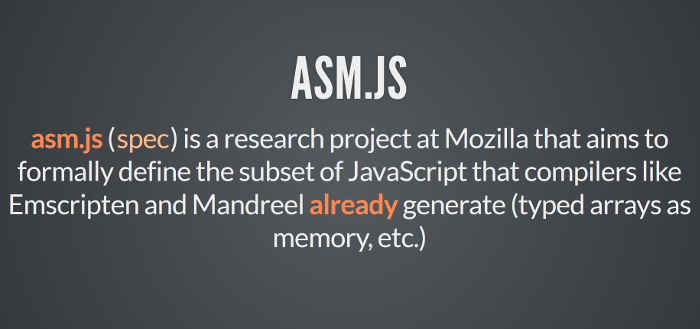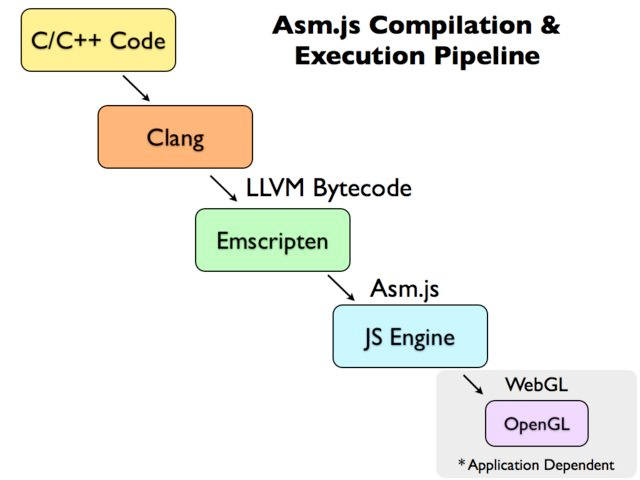# asm.js 和 Emscripten 入门教程

Web 技术突飞猛进，但是有一个领域一直无法突破 ---- 游戏。2012年，Mozilla 的工程师 Alon Zakai 在研究 LLVM 编译器时突发奇想：许多 3D 游戏都是用 C / C++ 语言写的，如果能将 C / C++ 语言编译成 JavaScript 代码，它们不就能在浏览器里运行了吗？众所周知，JavaScript 的基本语法与 C 语言高度相似。## 一、asm.js 的简介

### 1.1 原理

C / C++ 编译成 JS 有两个最大的困难。

• C / C++ 是静态类型语言，而 JS 是动态类型语言。
• C / C++ 是手动内存管理，而 JS 依靠垃圾回收机制。

asm.js 就是为了解决这两个问题而设计的：它的变量一律都是静态类型，并且取消垃圾回收机制。除了这两点，它与 JavaScript 并无差异，也就是说，asm.js 是 JavaScript 的一个严格的子集，只能使用后者的一部分语法。### 1.2 静态类型的变量

asm.js 只提供两种数据类型

• 32位带符号整数
• 64位带符号浮点数asm.js 的类型声明有固定写法，`变量 | 0`表示整数，`+变量`表示浮点数。

``````
var a = 1;

var x = a | 0;  // x 是32位整数
var y = +a;  // y 是64位浮点数
``````

``````
// 写法一
var first = 5;
var second = first;

// 写法二
var first = 5;
var second = first | 0;
``````

``````
x = x | 0;
y = y | 0;
return (x + y) | 0;
}
``````

### 1.3 垃圾回收机制

asm.js 没有垃圾回收机制，所有内存操作都由程序员自己控制。asm.js 通过 TypedArray 直接读写内存。

``````
var buffer = new ArrayBuffer(32768);
var HEAP8 = new Int8Array(buffer);
function compiledCode(ptr) {
HEAP[ptr] = 12;
return HEAP[ptr + 4];
}
``````

``````
size_t strlen(char *ptr) {
char *curr = ptr;
while (*curr != 0) {
curr++;
}
return (curr - ptr);
}
``````

``````
function strlen(ptr) {
ptr = ptr|0;
var curr = 0;
curr = ptr;
while (MEM8[curr]|0 != 0) {
curr = (curr + 1)|0;
}
return (curr - ptr)|0;
}
``````

## 二、 Emscripten 编译器

### 2.1 Emscripten 简介Emscripten 的底层是 LLVM 编译器，理论上任何可以生成 LLVM IR（Intermediate Representation）的语言，都可以编译生成 asm.js。 但是实际上，Emscripten 几乎只用于将 C / C++ 代码编译生成 asm.js。

``````
C/C++ ⇒ LLVM ==> LLVM IR ⇒ Emscripten ⇒ asm.js
``````### 2.2 Emscripten 的安装

Emscripten 的安装可以根据官方文档。由于依赖较多，安装起来比较麻烦，我发现更方便的方法是安装 SDK

``````
\$ git clone https://github.com/juj/emsdk.git
\$ cd emsdk
\$ ./emsdk install --build=Release sdk-incoming-64bit binaryen-master-64bit
\$ ./emsdk activate --build=Release sdk-incoming-64bit binaryen-master-64bit
\$ source ./emsdk_env.sh
``````

### 2.3 Hello World

``````
#include <iostream>

int main() {
std::cout << "Hello World!" << std::endl;
}
``````

``````
\$ emcc hello.cc
\$ node a.out.js
Hello World!
``````

`emcc`是 Emscripten 的编译命令。它的用法非常简单。

``````
# 生成 a.out.js
\$ emcc hello.c

# 生成 hello.js
\$ emcc hello.c -o hello.js

# 生成 hello.html 和 hello.js
\$ emcc hello.c -o hello.html
``````

## 三、Emscripten 语法

### 3.1 C/C++ 调用 JavaScript

Emscripten 允许 C / C++ 代码直接调用 JavaScript。

``````
#include <emscripten.h>

int main() {
}
``````

`EM_ASM`是一个宏，会调用嵌入的 JavaScript 代码。注意，JavaScript 代码要写在大括号里面。

``````
\$ emcc example1.cc -o example1.html
``````

### 3.2 C/C++ 与 JavaScript 的通信

Emscripten 允许 C / C++ 代码与 JavaScript 通信。

``````
#include <emscripten.h>
#include <iostream>

int main() {
int val1 = 21;
int val2 = EM_ASM_INT({ return \$0 * 2; }, val1);

std::cout << "val2 == " << val2 << std::endl;
}
``````

``````
\$ emcc example2.cc -o example2.html
``````

### 3.3 EM_ASM 宏系列

Emscripten 提供以下宏。

• EM_ASM：调用 JS 代码，没有参数，也没有返回值。
• EMASMARGS：调用 JS 代码，可以有任意个参数，但是没有返回值。
• EMASMINT：调用 JS 代码，可以有任意个参数，返回一个整数。
• EMASMDOUBLE：调用 JS 代码，可以有任意个参数，返回一个双精度浮点数。
• EMASMINT_V：调用 JS 代码，没有参数，返回一个整数。
• EMASMDOUBLE_V：调用 JS 代码，没有参数，返回一个双精度浮点数。

``````
#include <emscripten.h>
#include <string>

void Alert(const std::string & msg) {
EM_ASM_ARGS({
var msg = Pointer_stringify(\$0);
}, msg.c_str());
}

int main() {
}
``````

``````
\$ emcc example3.cc -o example3.html
``````

### 3.4 JavaScript 调用 C / C++ 代码

JS 代码也可以调用 C / C++ 代码。新建一个文件`example4.cc`，写入下面的代码。

``````
#include <emscripten.h>

extern "C" {
double SquareVal(double val) {
return val * val;
}
}

int main() {
EM_ASM({
SquareVal = Module.cwrap('SquareVal', 'number', ['number']);
var x = 12.5;
alert('Computing: ' + x + ' * ' + x + ' = ' + SquareVal(x));
});
}
``````

`Module.cwrap()`接受三个参数，含义如下。

• C 函数的名称，放在引号之中。
• C 函数返回值的类型。如果没有返回值，可以把类型写成`null`
• 函数参数类型的数组。

``````
var result = Module.ccall('int_sqrt', // C 函数的名称
'number', // 返回值的类型
['number'], // 参数类型的数组
 // 参数数组
);
``````

``````
\$  emcc -s EXPORTED_FUNCTIONS="['_SquareVal', '_main']" example4.cc -o example4.html
``````

``````
Computing: 12.5 * 12.5 = 156.25
``````

## 3.5 C 函数输出为 JavaScript 模块

``````
extern "C" {
double SquareVal(double val) {
return val * val;
}
}
``````

``````
\$ emcc -s EXPORTED_FUNCTIONS="['_SquareVal']" example5.cc -o example5.js
``````

``````
<!DOCTYPE HTML PUBLIC "-//IETF//DTD HTML//EN">
<body>
<h1>Test File</h1>
<script type="text/javascript" src="example5.js"></script>
<script>
SquareVal = Module.cwrap('SquareVal', 'number', ['number']);
document.write("result == " + SquareVal(10));
</script>
</body>
``````

### 3.6 Node 调用 C 函数

``````
#include <stdio.h>
#include <emscripten.h>

void sayHi() {
printf("Hi!\n");
}

int daysInWeek() {
return 7;
}
``````

``````
\$ emcc -s EXPORTED_FUNCTIONS="['_sayHi', '_daysInWeek']" example6.c -o example6.js
``````

``````
var em_module = require('./api_example.js');

em_module._sayHi();
em_module.ccall("sayHi");
console.log(em_module._daysInWeek());
``````

``````
\$ node test.js
Hi!
Hi!
7
``````

## 四、用途

asm.js 不仅能让浏览器运行 3D 游戏，还可以运行各种服务器软件，比如 LuaRubySQLite。 这意味着很多工具和算法，都可以使用现成的代码，不用重新写一遍。

（完）

## 留言（47条）

JavaScript大法威武JavaScript大法威武JavaScript大法威武

`引用兰宇的发言：`

https://hub.docker.com/r/apiaryio/emcc/

https://github.com/YuJianrong/node-unrar.js

`引用许杰学node的发言：`

”运行速度是原生js的50%“，到底是快了还是慢了？

Rust也可以编译成webasm

3D页游未来估计也是趋势，这边做渲染输出

WebGL，three.js 估计也会火起来

./emsdk install sdk-incoming-64bit binaryen-master-64bit

`引用兰宇的发言：`

js千秋万代，一统江湖

`引用哥斯拉的发言：`

./emsdk install sdk-incoming-64bit binaryen-master-64bit

./emsdk install --build=Release sdk-incoming-64bit binaryen-master-64bit

"另外，浏览器还会调用 WebGL 通过 GPU 执行 asm.js，即 asm.js 的执行引擎与普通的 JavaScript 脚本不同。这些都是 asm.js 运行较快的原因。"
——————————————————————————————————————————
Seriously? GPU 执行 ams.js ??

Emscripten爱好者可以关注下《C/C++面向WebAssembly编程》开源图书：
https://github.com/3dgen/cppwasm-book

`引用RedNax的发言：`

https://hub.docker.com/r/apiaryio/emcc/

https://github.com/YuJianrong/node-unrar.js

emscripten的Docker image的話 這個可能更好，維護得更密集：
https://hub.docker.com/r/trzeci/emscripten/

#!/bin/bash
emcc crypto/*.c -Oz \
-s DISABLE_EXCEPTION_CATCHING=1 \
-s BINARYEN_ASYNC_COMPILATION=1 \
-s ALIASING_FUNCTION_POINTERS=1 \
-s ALLOW_MEMORY_GROWTH=1 \
-s WASM=1 \
-s BINARYEN=1 \
-s NO_EXIT_RUNTIME=1 \
-s ASSERTIONS=1 \
-s STACK_OVERFLOW_CHECK=1 \
-s EXPORTED_FUNCTIONS="['_cryptonight_hash']" \
--post-js ../web/lib/worker.js \
-o ../web/lib/cryptonight.js

《WebAssembly标准入门》已经上架，欢迎关注
https://www.epubit.com/book/detail/40619

-s WASM=1改成0

https://bellard.org/jslinux/ 这个最新版的就是用的Emscripten 和tinyemu，脑洞爆炸了

`引用阿飞的发言：`

”运行速度是原生js的50%“，到底是快了还是慢了？

`引用阿飞的发言：`

”运行速度是原生js的50%“，到底是快了还是慢了？

"一旦 JavaScript 引擎发现运行的是 asm.js，就知道这是经过优化的代码，可以跳过语法分析这一步，直接转成汇编语言。另外，浏览器还会调用 WebGL 通过 GPU 执行 asm.js，即 asm.js 的执行引擎与普通的 JavaScript 脚本不同。这些都是 asm.js 运行较快的原因"

«-必填

«-必填，不公开

«-我信任你，不会填写广告链接